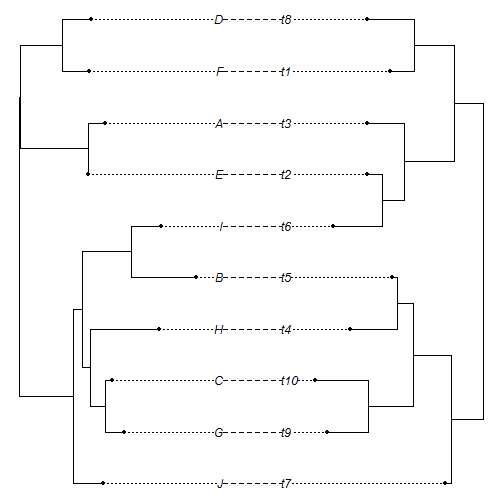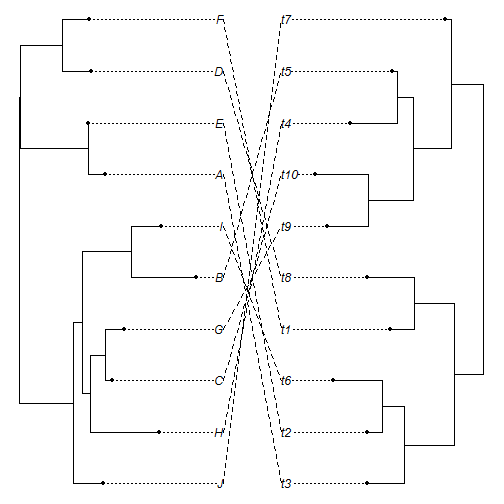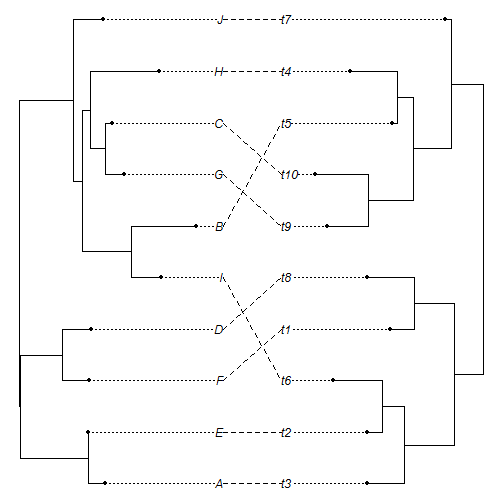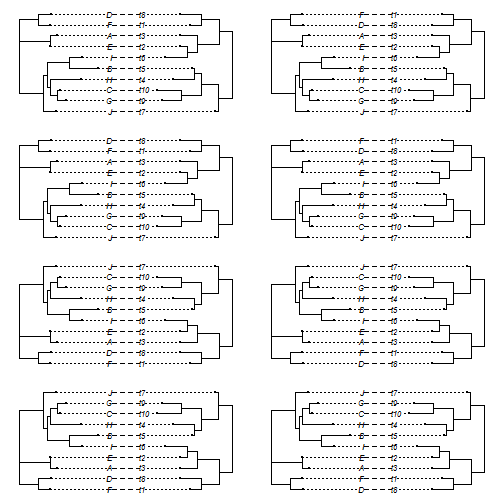## Wednesday, September 14, 2016

### New exhaustive search for `cophylo` method (and new object class)

I finally figured out how to perform an exhaustive search for tip matching in phytools co-phylogenetic plotting method, `cophylo`.

First, I wrote the function `allRotations` (described here) with the idea that if some rotation of the nodes existed such that the tips matched in their left-to-right ordering, then I should just be able to exhaustively rotate all the nodes of one tree, and amongst these, there should be a rotation to match the tips of the second tree. Seems logical, right?

Well, it turns out - not so. In fact, we have to rotate the nodes of both trees in all possible ways to guarantee that we find the best matching. For some reason, it has taken me a while to get this through my skull - so I was at times very perplexed as to why the previously mentioned approach didn't seem to work.

The update with this method can be seen here. I also added a new object class for a list of `"cophylo"` objects called a `"multiCophylo"` object, with its own S3 `print` and `plot` methods, in turn. The idea is that from an exhaustive search we will invariably find at least 2 node rotations of both trees that maximize matching (one & its mirror image). Sometimes we can find various such rotations, so I thought it would make sense to return all of the corresponding rotated `"cophylo"`, rather than arbitrarily choosing among them.

Note that the number of possible node rotations of a bifurcating tree with N tips is 2N-1 and the number of comparisons we thus have to make for two trees containg N1 and N2 tips, respectively is 2N1-1 × 2N2-1. These are all quite large quantities for even modestly sized phylogenies!

Here's a demo using relatively small phylogenetic trees.

First, load packages & simulate trees that do not share phylogenetic structure, but for which a tip matching exists:

``````library(phytools)
packageVersion("phytools")
``````
``````##  '0.5.51'
``````
``````set.seed(99)
N<-10
t1<-rtree(n=N,tip.label=LETTERS[1:N])
t2<-rtree(n=N)
assoc<-cbind(t1\$tip.label,t2\$tip.label)
assoc
``````
``````##       [,1] [,2]
##  [1,] "J"  "t7"
##  [2,] "G"  "t9"
##  [3,] "C"  "t10"
##  [4,] "H"  "t4"
##  [5,] "B"  "t5"
##  [6,] "I"  "t6"
##  [7,] "E"  "t2"
##  [8,] "A"  "t3"
##  [9,] "F"  "t1"
## [10,] "D"  "t8"
``````
``````plot(cophylo(t1,t2,assoc=assoc))
``````
``````## Rotating nodes to optimize matching...
## Done.
``````Now, let's rotate the crap out of both trees and show that with our optimization algorithm, we do not find the matching:

``````for(i in 1:100) t1<-rotateNodes(t1,node=sample(1:t1\$Nnode+Ntip(t1),1))
for(i in 1:100) t2<-rotateNodes(t2,node=sample(1:t2\$Nnode+Ntip(t2),1))
## first unrotated
plot(cophylo(t1,t2,assoc=assoc,rotate=FALSE))
````````````## then rotated with our optimization routine
obj<-cophylo(t1,t2,assoc=assoc)
``````
``````## Rotating nodes to optimize matching...
## Done.
``````
``````plot(obj)
``````Finally, let's try the new exhaustive search method:

``````obj<-cophylo(t1,t2,assoc=assoc,methods="exhaustive")
``````
``````## Rotating nodes to optimize matching...
## Done.
``````
``````obj
``````
``````## Object of class "multiCophylo" containg 8 objects of class "cophylo".
``````
``````par(mfrow=c(4,2))
plot(obj,mar=rep(1.1,4))
``````That's it. Note that this is not expected to work for trees that are not perfectly bifurcating (although our optimization algorithm does, with `rotate.multi=TRUE`).